NCERT Questions

Class 9
Chapter 12 Class 9 - Sound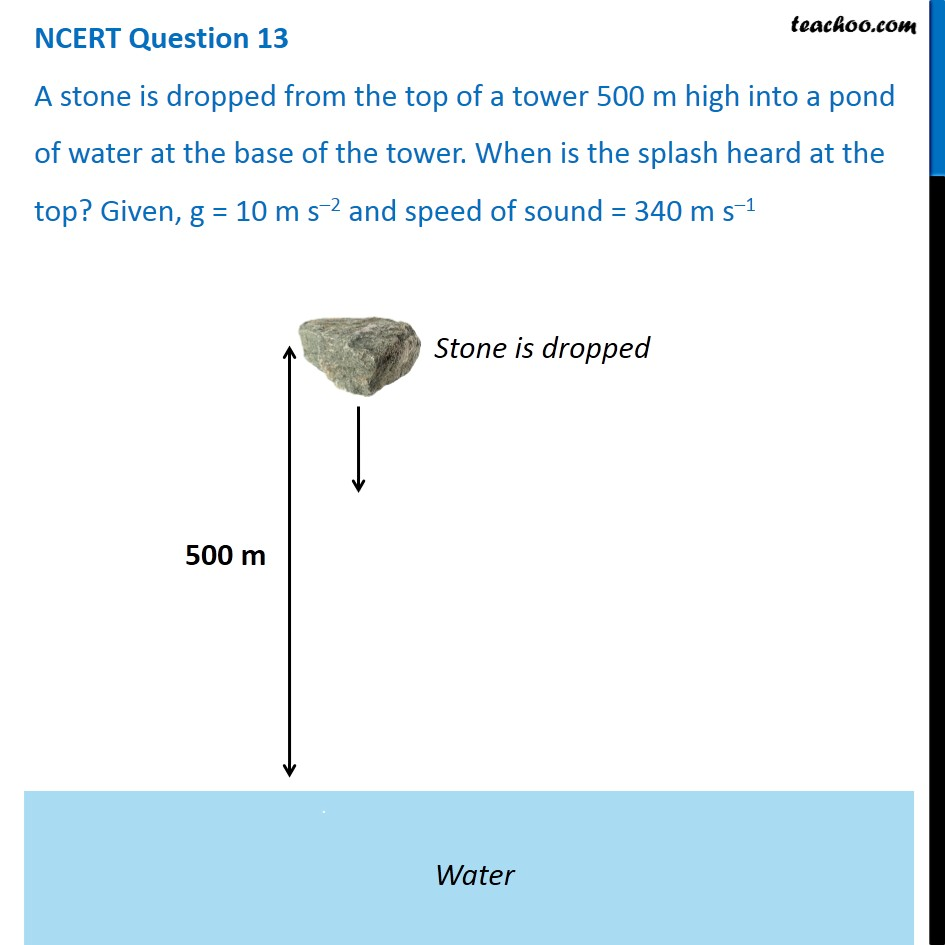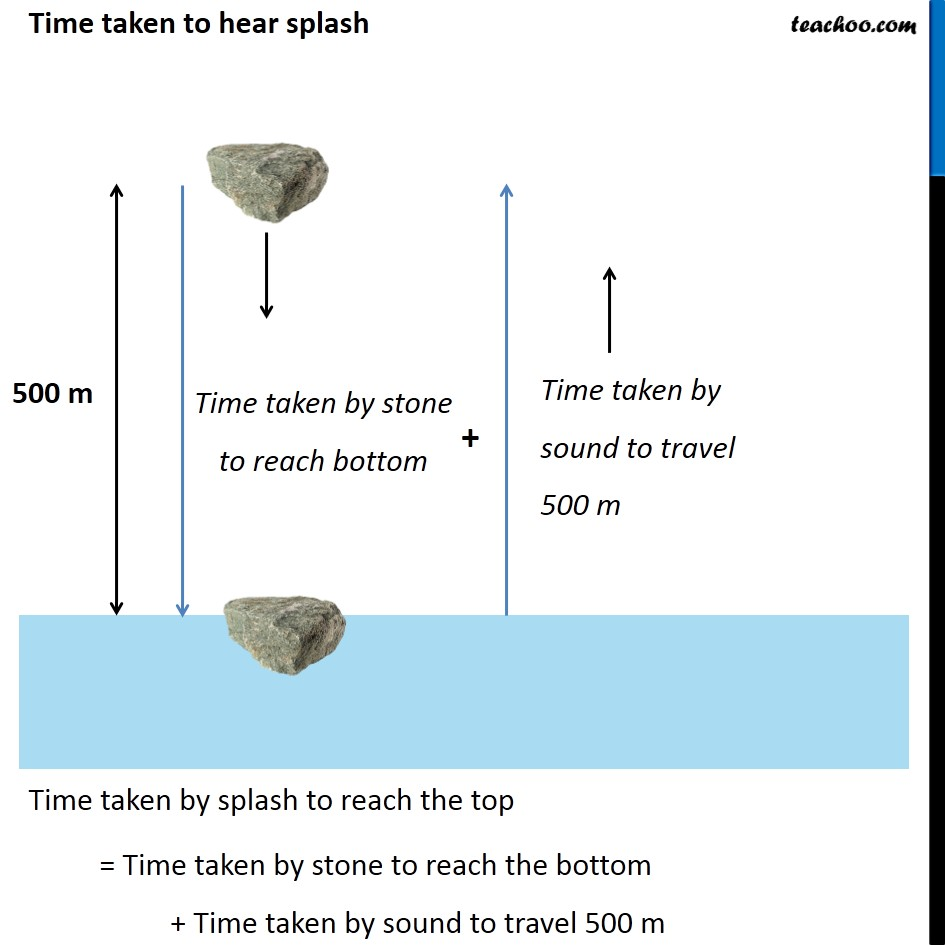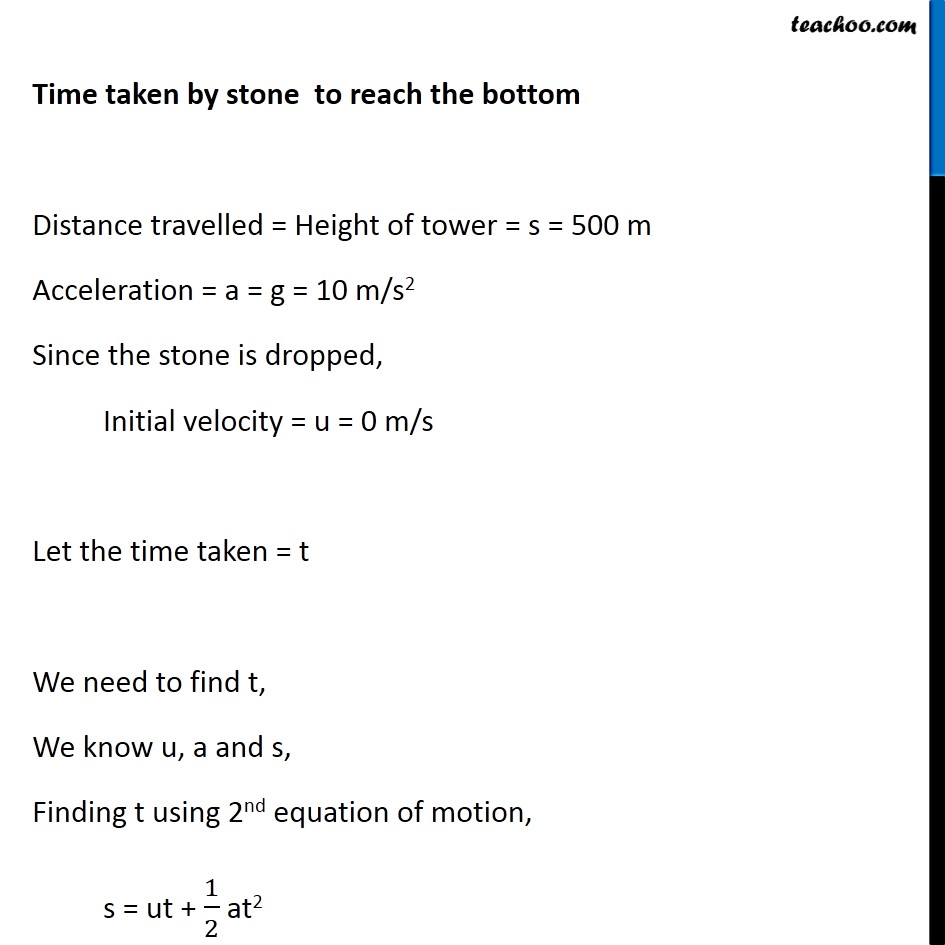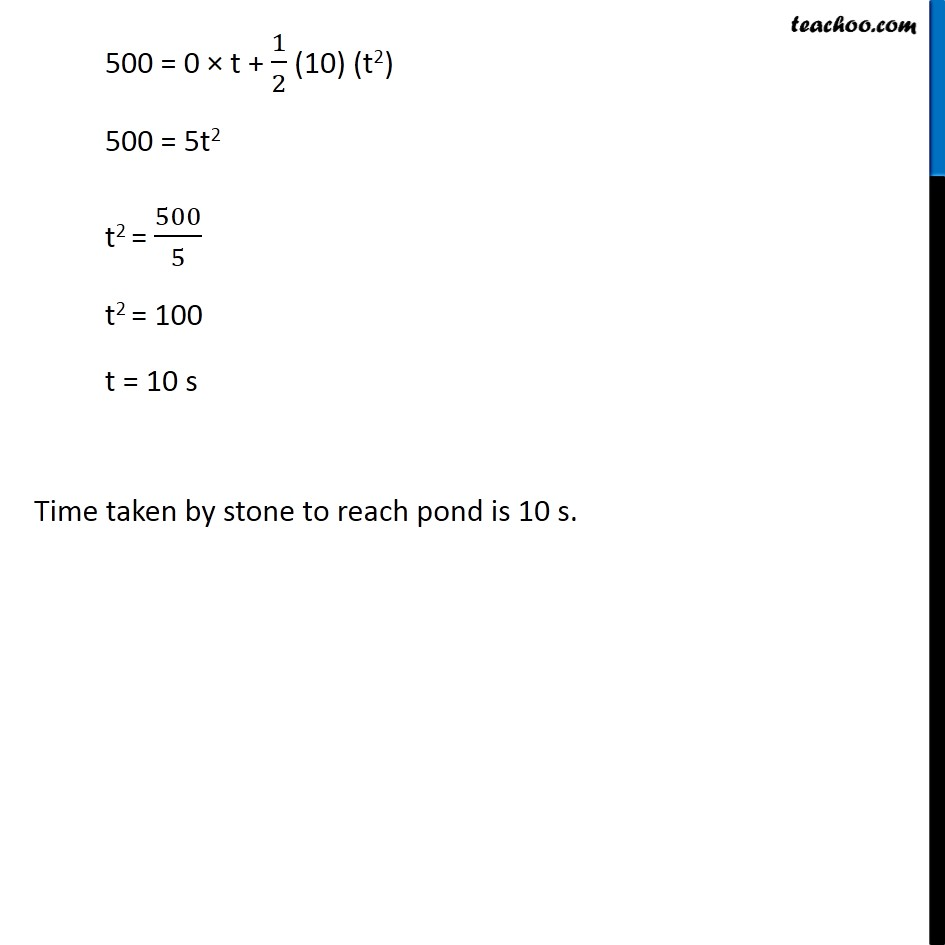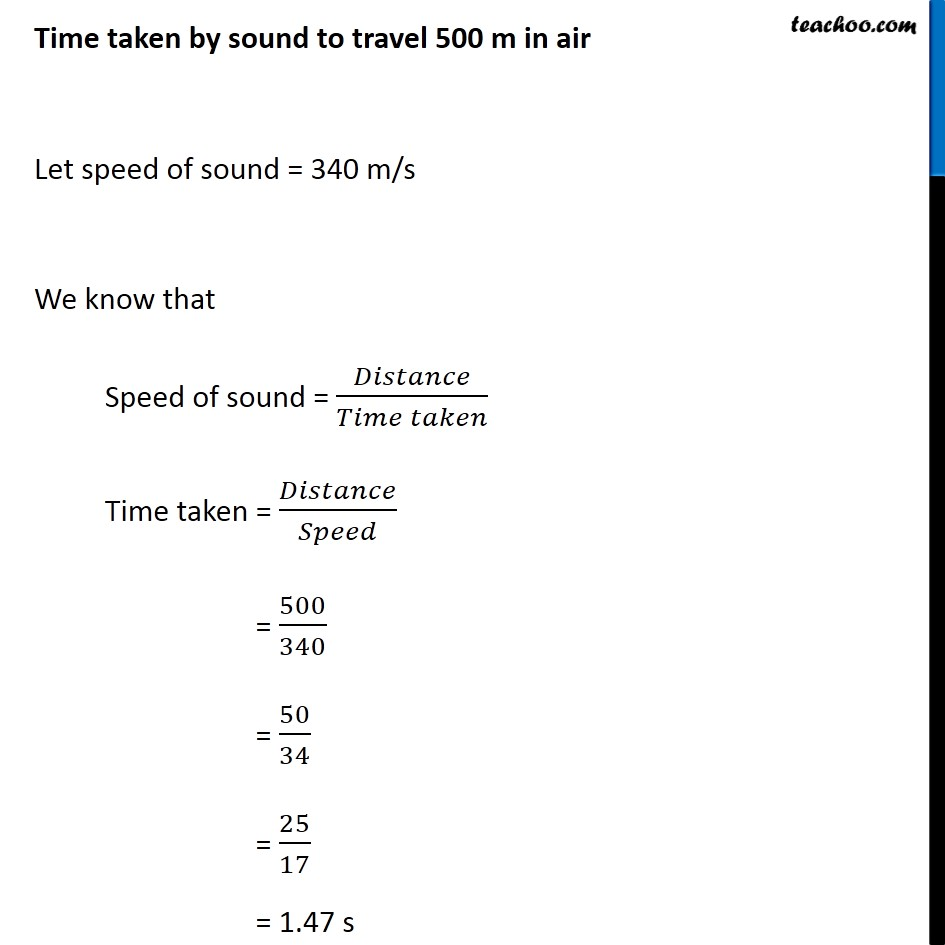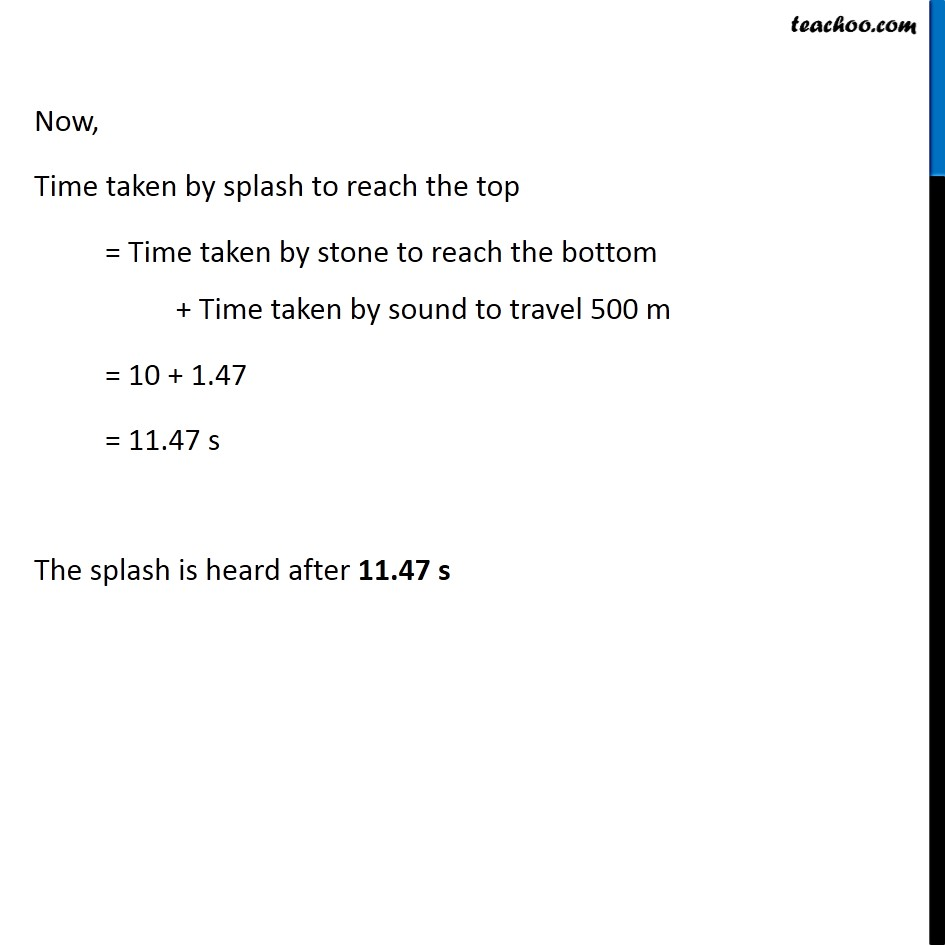Learn in your speed, with individual attention - Teachoo Maths 1-on-1 Class

### Transcript

NCERT Question 13 A stone is dropped from the top of a tower 500 m high into a pond of water at the base of the tower. When is the splash heard at the top? Given, g = 10 m s–2 and speed of sound = 340 m s–1 Time taken to hear splash Time taken by splash to reach the top = Time taken by stone to reach the bottom + Time taken by sound to travel 500 m Time taken by stone to reach the bottom Distance travelled = Height of tower = s = 500 m Acceleration = a = g = 10 m/s2 Since the stone is dropped, Initial velocity = u = 0 m/s Let the time taken = t We need to find t, We know u, a and s, Finding t using 2nd equation of motion, s = ut + 1/2 at2 500 = 0 × t + 1/2 (10) (t2) 500 = 5t2 t2 = 500/5 t2 = 100 t = 10 s Time taken by stone to reach pond is 10 s. Time taken by sound to travel 500 m in air Let speed of sound = 340 m/s We know that Speed of sound = 𝐷𝑖𝑠𝑡𝑎𝑛𝑐𝑒/(𝑇𝑖𝑚𝑒 𝑡𝑎𝑘𝑒𝑛) Time taken = 𝐷𝑖𝑠𝑡𝑎𝑛𝑐𝑒/𝑆𝑝𝑒𝑒𝑑 = 500/340 = 50/34 = 25/17 = 1.47 s Now, Time taken by splash to reach the top = Time taken by stone to reach the bottom + Time taken by sound to travel 500 m = 10 + 1.47 = 11.47 s The splash is heard after 11.47 s